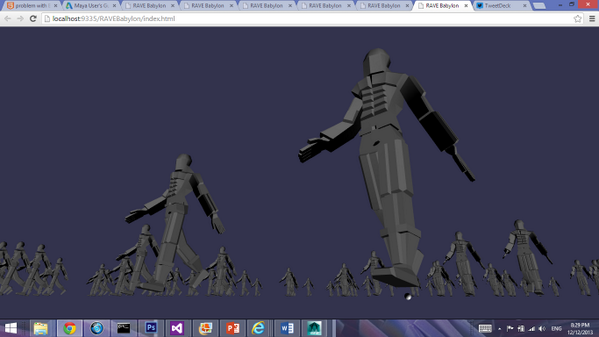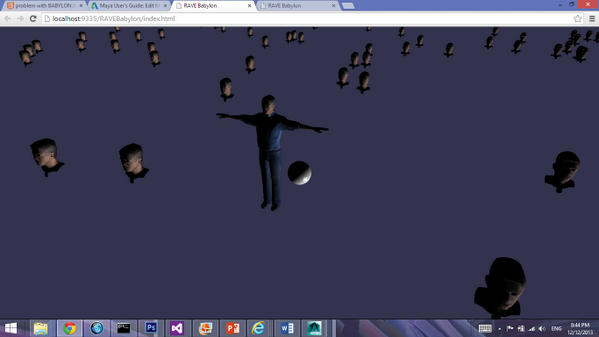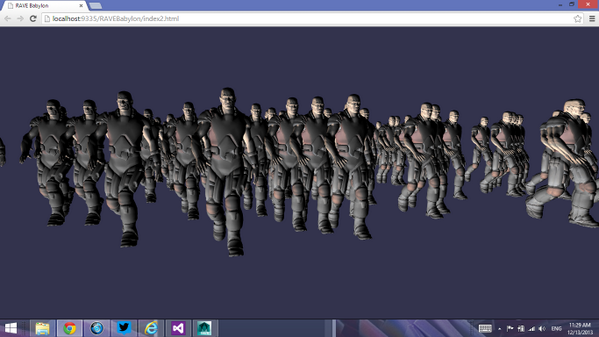# How do you clone more complex models?

## Recommended Posts

I can get clone to work with a simple model (only 2 Meshes)But with a more complex model that has submeshes like heads, torsos, and other elements, I can't get Mesh.Clone to work except on individual submeshes:This also happens when I try to clone dude.fbx

Am I using clone incorrectly? Here's the code I used to do the above (no animation)

myavatar = BABYLON.SceneLoader.ImportMesh("FlorSkin", "", "FlorAvatar.babylon", scene, function (newMeshes, particleSystems, skeletons) {
a = newMeshes;
a.position = new BABYLON.Vector3(0, 0,5);
for (var i = 0; i < 100; i++) {
var r = newMeshes.clone("r" + i); // 0-crashes, 1-clones eyeballs, 4-clones the head
r.position = new BABYLON.Vector3(Math.floor(Math.random() * 101) - 50, 0, Math.floor(Math.random() * 101) - 50);
}

});

Thank you.

##### Share on other sites

You should be aware that some meshes (like the 0 in your case) should have no geometry (they are only invisible impostors)

Could you share the error you have with mesh ID=1 or 2 ?

##### Share on other sites

Thanks for the comment. Yes, I quickly discovered that mesh ID=0 had nothing to clone.

mesh ID =1, 2, 3, etc. don't crash, but if I use mesh ID=1 it only clones a portion of the model.  For example, in my second image meshID=1 would only clone the eyeball.

This happens with the Microsoft dude.fbx model too. If you try to clone with mesh ID=1, you only get the chest area.  I will try to recreate with dude.fbx to show you (after I finish my morning writings :-)

##### Share on other sites

This, should work.

`myavatar = BABYLON.SceneLoader.ImportMesh("FlorSkin", "", "FlorAvatar.babylon", scene, function (newMeshes, particleSystems, skeletons) {    a = newMeshes;    a.position = new BABYLON.Vector3(0, 0, 5);    for (var i = 0; i < 100; i++) {                for(var a = 1; a < newMeshes.length; a++) {            r = newMeshes[a].clone("r_" + i + "_" + a);            var pos = Math.floor(Math.random() * 101) - 50;            r.position = new BABYLON.Vector3(pos, 0, pos);        }     }    });`
##### Share on other sites

I have change the code which I show above which should be better to regulate the position of every element of a model.

`myavatar = BABYLON.SceneLoader.ImportMesh("FlorSkin", "", "FlorAvatar.babylon", scene, function (newMeshes, particleSystems, skeletons) {    a = newMeshes;    a.position = new BABYLON.Vector3(0, 0, 5);        for (var i = 0; i < 100; i++) {           var r = new Array();             var pos = Math.floor(Math.random() * 101) - 50;        for(var a = 1; a < newMeshes.length; a++) {            r[a - 1] = newMeshes[a].clone("r_" + i + "_" + a);        }                for(var o = 1; o < r.length; o++) {            r[o].position = new BABYLON.Vector3(pos, 0, pos);        }    }    });`

to test

##### Share on other sites

Thanks Guys! I ended up doing this and trying it on Dude.  Is this the right way to do it (your previous example didn't have animation)

myavatar = BABYLON.SceneLoader.ImportMesh("him", "Dude/", "dude.babylon", scene, function (newMeshes, particleSystems, skeletons) {
myava = newMeshes;
a = myava;
a.position = new BABYLON.Vector3(0, 0, 5);
scene.beginAnimation(skeletons, 0, 120, 1.0, true);

dudes = [];
for (i = 0; i < 100; i++) {
var xrand = Math.floor(Math.random() * 501) - 250;
var zrand = Math.floor(Math.random() * 501) - 250;
var c = [];
for (j = 1; j < newMeshes.length; j++) {
c[j] = newMeshes[j].clone("c" + j);
c[j].position = new BABYLON.Vector3(xrand, 0, zrand);
c[j].skeleton = newMeshes[j].skeleton.clone();
scene.beginAnimation(c[j].skeleton, 0, 120, 1.0, true);
}
dudes = c;
}##### Share on other sites

Haha. Anyway, I just cleaned up the code slightly and added two short comments for the community

myavatar = BABYLON.SceneLoader.ImportMesh("him", "Dude/", "dude.babylon", scene, function (newMeshes, particleSystems, skeletons) {
newMeshes.position = new BABYLON.Vector3(0, 0, 5);  // The original dude
scene.beginAnimation(skeletons, 0, 120, 1.0, true);

dudes = [];
for (i = 0; i < 10; i++) { // 10 clones
var xrand = Math.floor(Math.random() * 501) - 250;
var zrand = Math.floor(Math.random() * 501) - 250;
var c = [];
for (j = 1; j < newMeshes.length; j++) {
c[j] = newMeshes[j].clone("c" + j);
c[j].position = new BABYLON.Vector3(xrand, 0, zrand);
c[j].skeleton = newMeshes[j].skeleton.clone();
scene.beginAnimation(c[j].skeleton, 0, 120, 1.0, true);
}
dudes = c;
}

## Join the conversation

You can post now and register later. If you have an account, sign in now to post with your account.
Note: Your post will require moderator approval before it will be visible.×   Pasted as rich text.   Paste as plain text instead

Only 75 emoji are allowed.